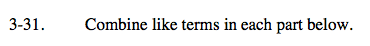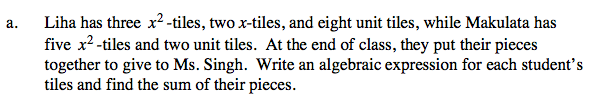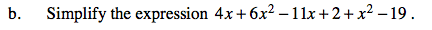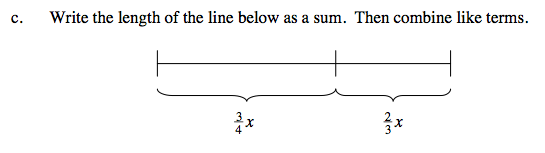### Home > CAAC > Chapter 3 > Lesson 3.1.3 > Problem3-31

3-31.Write down Liha's and Makulata's tile pieces as expressions.

Liha 3x2 + 2x + 8
Makulata 5x2 + 2

3x2 + 2x + 8 + 5x2 + 2 = 8x2 + 2x + 10Add like terms and then simplify.Write the two terms down as an expression.

$\frac{3}{4}{\it x} + \frac{2}{3}{\it x}$

Convert both fractions to a common denominator before combining like terms.